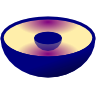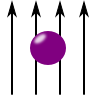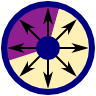# Capacitors & Co - Dielectrics

The step from the microscopical to the macroscopical Maxwell equations is as fundamental as it is often underestimated. In these problems we will make ourself familiar with the electrostatic version of these equations. We will assume that the electric field induces polarization charges $$\rho_\mathrm{pol}$$ in a material as the sources of a polarization density $$\mathbf{P}$$,$\begin{eqnarray*} \varepsilon_{0}\nabla\cdot\mathbf{E}\left(\mathbf{r}\right)=\rho\left(\mathbf{r}\right)&=&\rho_{\mathrm{ext}}\left(\mathbf{r}\right)+\rho_{\mathrm{pol}}\left(\mathbf{r}\right)\\&=&\rho_{\mathrm{ext}}\left(\mathbf{r}\right)-\mathrm{div}\mathbf{P}\left(\mathbf{r}\right)\ , \end{eqnarray*}$such that we are able to introduce a new field, the electric displacement field $$\mathbf{D}$$:$\begin{eqnarray*} \nabla\cdot\left[\varepsilon_{0}\mathbf{E}\left(\mathbf{r}\right)+\mathbf{P}\left(\mathbf{r}\right)\right]&\equiv&\nabla\cdot\mathbf{D}\left(\mathbf{r}\right)\\&=&\rho_{\mathrm{ext}}\left(\mathbf{r}\right) \ . \end{eqnarray*}$Here, $$\rho_\mathrm{ext}$$ corresponds to all charges that do not correspond to polarization charges. Using the boundary conditions we can derive from these equations will help us to determine the electrostatic properties of certain dielectric bodies. A special attention will be made to capacitors as these are devices we will be able to describe thoroughly.

Important quantities to characterize dielectric materials are the (relative) permittivity $$\varepsilon$$ and the suszeptibility $$\chi$$. Both are introduced for linear materials as follows:$\begin{eqnarray*} \mathbf{D}\left(\mathbf{r}\right)&=&\varepsilon_{0}\mathbf{E}\left(\mathbf{r}\right)+\mathbf{P}\left(\mathbf{r}\right)\\&=&\varepsilon_{0}\mathbf{E}\left(\mathbf{r}\right)+\varepsilon_{0}\chi\left(\mathbf{r}\right)\mathbf{E}\left(\mathbf{r}\right)\\&=&\varepsilon_{0}\varepsilon_{r}\left(\mathbf{r}\right)\mathbf{E}\left(\mathbf{r}\right)\equiv\varepsilon\left(\mathbf{r}\right)\mathbf{E}\left(\mathbf{r}\right)\ . \end{eqnarray*}$

## Spherical Capacitor with Varying PermittivityIn general, capacitance calculations can be quite cumbersome involving complicated integrals. Whenever symmetries are present, we may find the capacitances much easier. Learn in this problem how to determine the properties of a spherical capacitor with a varying parmittivity of the dielectric.

## A Dielectric Sphere in a Homogeneous Electric FieldIn this problem we will encounter the main physical features of dielectric spheres - their induced field and polarizability. The result is of great interest: understanding the interaction of light with small particles is one of the main concerns of nanophotonics. It leads to astonishing applications like cloaking, improvement of solar cells and lithography with extreme resolution.

## An Electric Charge in front of a Dielectric InterfaceThe method of image charges can also be applied to problems including dielectrics. In this problem, a charge in front of an interface between two dielectrics is considered. Find out how to solve it and what happens if the charge is put in between the dielectrics.

## The Coaxial Capacitor with Two Embedded DielectricsModern electronic devices are made of certain fundamental building blocks, for example capacitors. Understanding their properties is with respect to their geometrical parameters is of great importance particularly in electrical engineering. In this problem we will calculate the features of a coaxial capacitor that contains two different media.